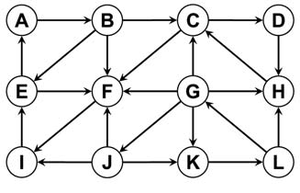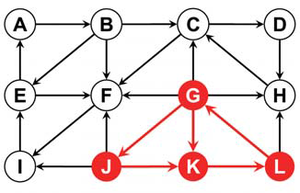# Model Checking for Hybrid Systems

## Model Checking for Hybrid Systems

The behavior of many technical devices can be described by specifying their states and state transitions. A washing machine can, for example, be in the starting state “door open, drum empty, machine turned off” or the state “washing.” State transitions are changes from one state to another. These can take place automatically, such as the change between washing and drainage, or through other actions, for example when a button is pressed. There are, however, possible states that are obviously undesirable, for example: “water inlet open, door open.” If we want to verify that the machine is safe, then we must show that there is no sequence of state transitions leading from the starting state to such an unsafe state.

How can this problem be approached? We can describe states and state transitions using graphs. To this end, we symbolize each state by a circle and each possible state transition by an arrow. The result may look as follows:##### example

Let us assume that A is the starting state and L is unsafe. What are the predecessor states of L, i.e., the states from which an arrow points to L? Obviously, there is only one such state: K. K is thus also unsafe, since if K can be reached, then L can be reached from there. K’s predecessor states, namely G and J, are unsafe for the same reason. If we take a look at the predecessor states of G and J (namely L and G), we can see that these are already known to be unsafe. This means that there is no way of reaching the state set {G, J, K, L} from outside.Non-safe states

It is therefore clear that {G, J, K, L} are the only states that are unsafe per se, or from which an unsafe state can be reached. All other states, in particular the starting state A, are safe. This technique for verifying the safety of a system is known as model checking.

Unfortunately, the number of states can quickly lead to problems in practice. Imagine that the behavior of an electronic device depends on 60 bits (b1,..., b60), i.e., 60 components that can each have the value of true or false. Now, 60 bits are not many, but if all the possible combinations are taken into account, we arrive at 260 states, more than one quintillion. Symbolic model checking offers a way out here: We no longer enumerate the unsafe states and permissible state transitions explicitly, but we represent them symbolically using logical formulas. For example, the formula

b5 and not b32

describes all states for which b5 is true and b32 is false – this is a quarter of a quintillion states, which would otherwise have to be enumerated explicitly.

A new problem emerges if we try to verify the safety of a vehicle control system in this way. Such a control system is a hybrid system. This means that one is no longer dealing solely with discrete state changes (e.g., acceleration or braking), but also with variables such as speed, which can change continuously. The state formulae must therefore also include numerical variables, for example:

b5 and not b32 and (x2 > 50).

Discrete state transitions can still be computed in the same way as for purely discrete systems. What about the continuous state transitions? As long as the dynamic behavior is simple from a mathematical point of view (e.g., if the speed changes uniformly), one can use a method known as quantifi er elimination. Certain technical tricks are needed, however, to prevent arriving at too large a formula too quickly. In our work group, we are currently focusing on developing methods that can be applied even to more complicated dynamic behaviors.

## Uwe WaldmannRG. 1 Automation of Logic
Phone
+49 681 9325-2905
Email
uwe@mpi-inf.mpg.de